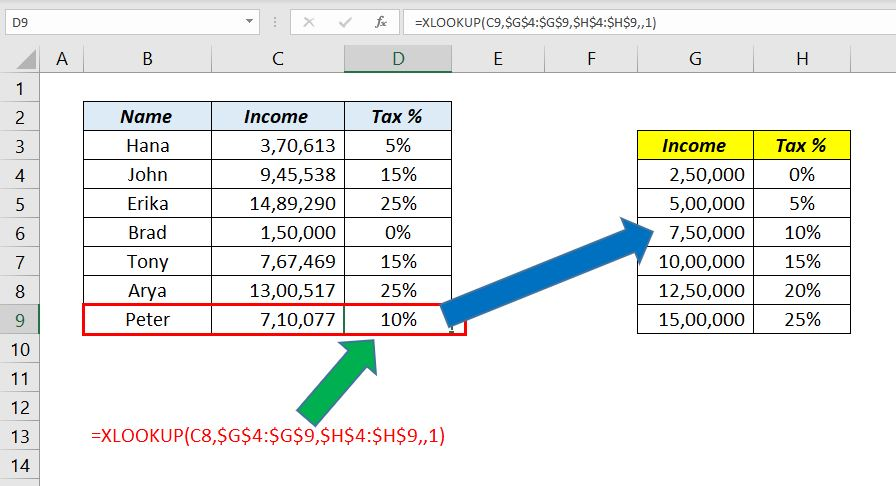# 7 Reasons Why XLOOKUP function is Superior to VLOOKUP functionIn this blog post, I will explain the reasons Why the XLOOKUP function in Excel is Superior to the VLOOKUP function.

#### 1. XLOOKUP function returns an Exact match by default

The default value returned by the XLOOKUP function is an Exact Match. With 3 arguments, XLOOKUP can return an exact match and this makes XLOOKUP easy to use than VLOOKUP or INDEX+MATCH.

VLOOKUP function always looks for an approximate match unless we use FALSE in the place of the fourth argument. For an exact match, the combination of INDEX/MATCH functions needs 5 arguments.

#### 2. XLOOKUP function can return multiple values

XLOOKUP function is an Array function and can return values into multiple cells. VLOOKUP function or the combination of INDEX-MATCH functions return a single value into a single cell.

#### 3. XLOOKUP function can perform lookups into the left side of the dataset

VLOOKUP function is designed to search for a value in the first column of a table and perform a lookup on the right side of that table. But the XLOOKUP function can search for a matching value on the right side as well as the left side of a Table.

Anyway, INDEX + MATCH combo is at par with XLOOKUP in this regard.

#### 4. XLOOKUP function can return a Custom value if matching values aren’t found

VLOOKUP function, as well as the INDEX+MATCH return a #N/A error if a matching value isn’t found. IFERROR function is generally used to trap this error.

But if you are using XLOOKUP function, there is no need to wrap XLOOKUP function with IFERROR function for an expected error. The fourth argument if_not_found of the XLOOKUP function is designed to return a custom value when the function cannot find a matching value.

In the following example, the 4th argument if_not_found of XLOOKUP function is used to return the text ‘Employee not found’ if the function can’t find a matching value.

#### 5. XLOOKUP function can search Bottom to Top of a dataset

VLOOKUP Function searches from Top to Bottom of an array while XLOOKUP function can search from Top to Bottom as well as from Bottom to Top in the lookup_array.

In the following example, XLOOKUP function is used to find the salesperson who made the last sales on the date 10-02-2020. When the value -1 (Search last to first) is used as the 6th argument, XLOOKUP function will search from the bottom of the table and returns the name of the salesperson who made the last sale on 10-02-2020.

#### 6. XLOOKUP function can return the Next Largest Item

VLOOKUP function always returns the next smallest item where XLOOKUP function can return the next smallest item as well as the next largest item. The 5th argument in XLOOKUP function match_mode is designed for this purpose. match_mode has 4 options and the third option is for returning an Exact match or the Next Larger Item.

In the following example, XLOOKUP function is used to find Tax % according to income of an individual. When the value -1 (Exact match or next larger item) is used as the 5th argument, XLOOKUP function will return a value or values against the exact match of the income or the next larger amount.

#### 7. XLOOKUP function can return the First and Last match of the lookup value

Using the 6th argument, match_mode of XLOOKUP function, the first and last matches of the lookup_value can be found out.

In the following example, XLOOKUP function returns the corresponding value against the first matching value, provided the values in the lookup_array are sorted in Alphabetical order.

Another example in which XLOOKUP function returns the corresponding value against the last matching value provided the values in the lookup_array are sorted in Reverse Alphabetical order.

More about Excel Formulas and Functions